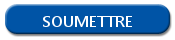# Load a package in R

The R programming language has many libraries. Each library (or package) has a set of functions dedicated to a specific use: date manipulation, dataset or character processing…

However, all the libraries aren't loaded when we launch R, so we haves to call some specific libraries in our scripts. For that, R have 2 functions: library() and require().

## Load an R package with library()

The function library() is the most used function to load a package in R. Its use is very easy: you just have to write the name of the library you want to load.

For example, to call the library DPLYR, you have to write the following instruction:

`library(dplyr)`

If the library is available, it will be loaded. Otherwise, the script will stop. Be careful, the function library() is case sensitive. Moreover, as the loaded library is an object, it doesn't need the use of quotation marks.

## Call an R package with the function require()

You can load libraries with the function require(). Using of this function is the same as the function library(). For example, to load the function DPLYR, you have to write the following instruction:

`require(dplyr)`

So, what is the difference between library() and require()?

The function require() is adapted to be used in a function and send an answer in the form of a Boolean variable.

If the package is loaded when the function require() is executed, the function send TRUE.

If the package isn't loaded, the function will send a FALSE variable. The script isn't stopped, and the developer can choose which action to realize if the function isn't loaded.